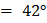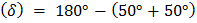# A ray of light travelling from glass to air (refractive index of glass=1.5). The angle of incidence isThe deviation of the ray is a)b)c)d)## Question ID - 152634 :- A ray of light travelling from glass to air (refractive index of glass=1.5). The angle of incidence isThe deviation of the ray is a)b)c)d)3537

 (b) Refractive index,Critical angle for glassWhen the angle of incidence in the denser medium is greater than the critical angle, reflection takes place inside the denser medium. Hence, a ray of light incident atin glass medium undergoes total internal reflection. Deviation(from the figure) OrRelation between critical angles of water and glass is a)b)c)d)